# Rotate Polygon using Attribute

680
3
05-07-2021 12:13 AM
Labels (1)New Contributor II

Good Morning

I am trying to rotate feature class with over 700 polygons based on the angle in an attribute? These polygons are all based on center points that were buffered and then run the create envelope tool. All the polygons now are oriented N/S. Any wok flows would be appreciated.

Thanks

Bruce Burwell

Tags (4)
3 Repliesby
New Contributor III

Hello,

Try using arcpy, see the code below.

``````def rotation(rotatefield):
def rotatepoint(point, pivotpoint, angle):
ox, oy = pivotpoint.X, pivotpoint.Y
px, py = point.X, point.Y
qx = ox + math.cos(angle_rad) * (px - ox) - math.sin(angle_rad) * (py - oy)
qy = oy + math.sin(angle_rad) * (px - ox) + math.cos(angle_rad) * (py - oy)
return arcpy.Point(qx,qy)

with arcpy.da.UpdateCursor("annotation_polygons",['SHAPE@', rotatefield]) as cursor:
for row in cursor:
polylist = []
for part in row:
partlist = []
for pnt in part:
if pnt is not None:
partlist.append(rotatepoint(pnt, arcpy.Point(row.X, row.Y), row))
polylist.append(partlist)
row = arcpy.Polygon(arcpy.Array(polylist))
cursor.updateRow(row)``````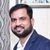Occasional Contributor II

Hi Anyry,

Here is the solution

Step 1: Polygon with Attribute Values. I have created a field name called "Angle" and manually keeping some values. (Refer Screenshot)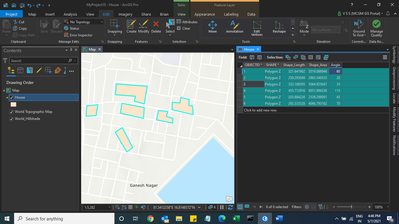Step 2: Go to the insert tab and click the new notebook

Step 3: Paste the script, which I have attached in mentioned in notepad and attached. (Refer Screenshot)Step 4: make changes in two placed feature class name and attribute field name. Refer screenshot.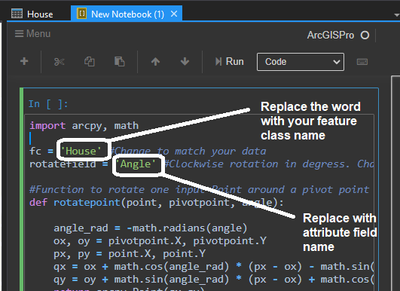Step 5: Hit the Run button in a notebook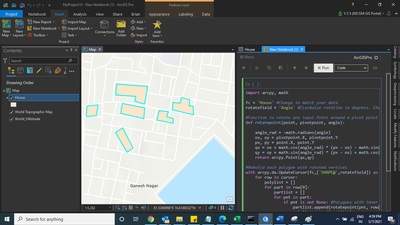Step 6: Here is the output results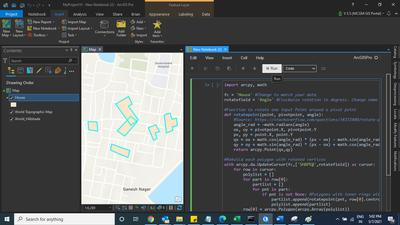Enjoy.....by Anonymous User
Not applicable

This throws a NoneType is not iterable error.